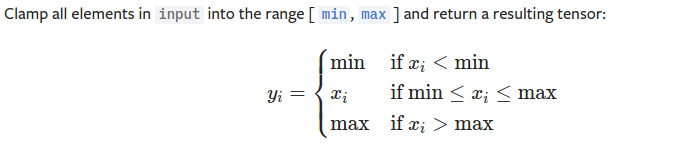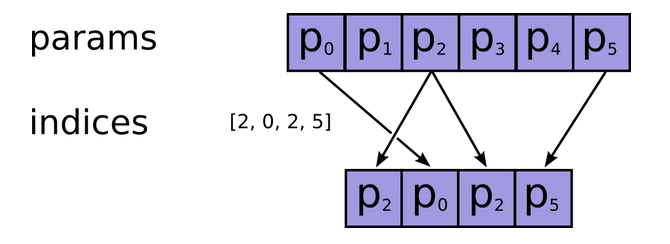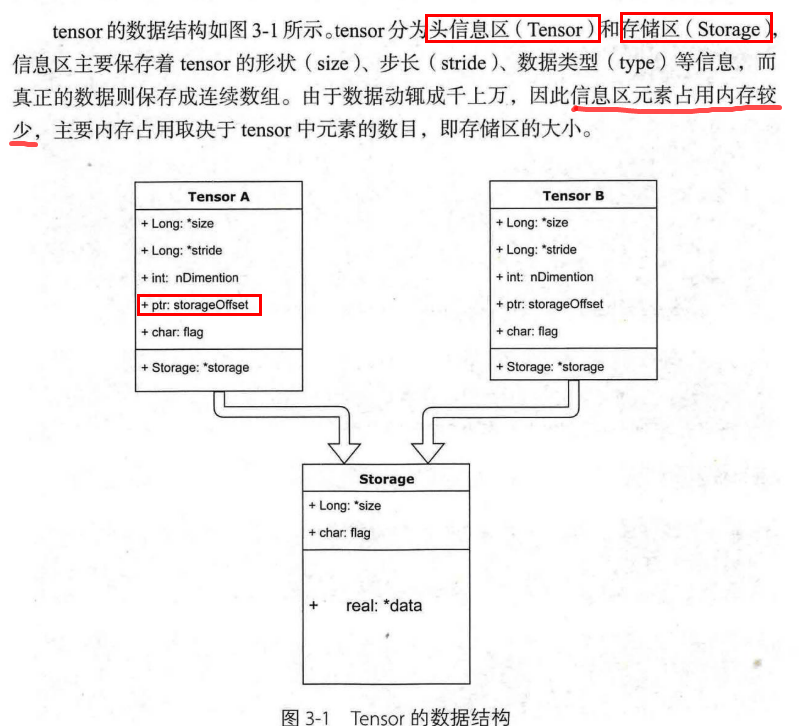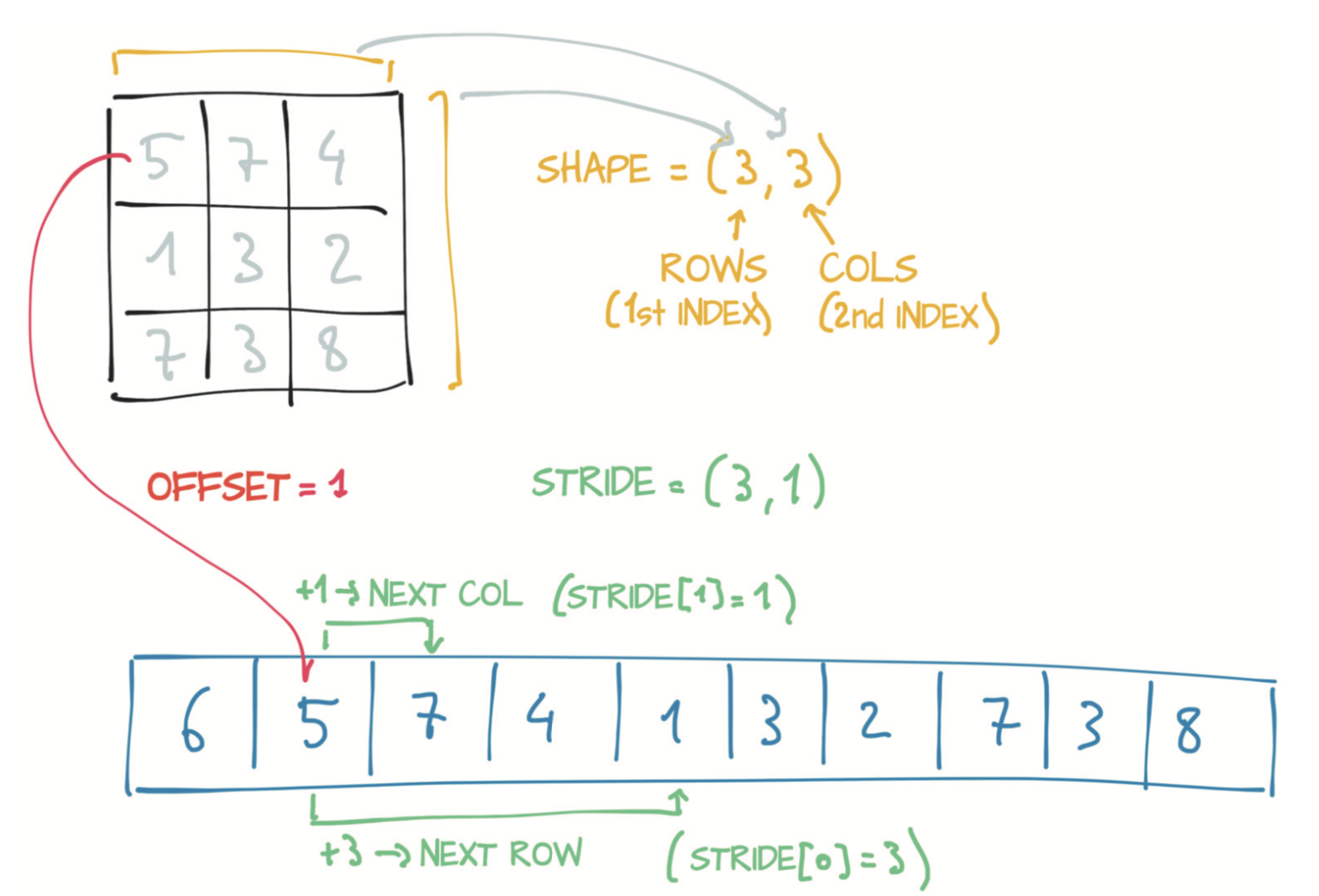# Pytorch 中的张量系统：Tensor

## 一、Torch 简介

### 1、Torch 最重要的特点及功能

• N 维数组对象(tensor)：该对象是存储单一数据类型的多维数组（可表示标量、向量、矩阵或张量等）
• 具有矢量运算和复杂广播能力，内存使用效率高
• 具有线性代数、随机数生成以及傅里叶变换功能

### 2、Torch 与 Numpy 的区别与联系

• Numpy 定位是各种各样的科学计算，其在 CPU 上比较快
• TF 和 Torch 等的定位是机器学习，它们在 Numpy 的基础上做了扩展，使其支持 GPU 编程、分布式编程、自动微分等特性
• torch==numpy, tensor==ndarray：torch is a Tensor library like NumPy, with strong GPU support

## 二、Tensor 使用

### 1. tensor 的创建

• 通过构造函数创建稠密矩阵和稀疏矩阵

• torch.tensor(data, dtype=None, device=None, requires_grad=False, pin_memory=False)
• data： array_like，为 python 列表(list of list)、元组、数组、标量或其它序列类型
• dtype： torch.float32、torch.int32、torch.bool
• device： torch.device('cpu')、'cpu'；cuda1=torch.device('cuda:1')、'cuda:1'、1
• requires_grad： 是否需要求解梯度，默认 False
• torch.sparse_coo_tensor(indices, values, size=None, dtype=None, device=None, requires_grad=False)
• indices： array_like，shape 为 2×N，N为非零元素的数量，第一维为横坐标，第二维为纵坐标
• values： array_like，非零元素的填充值
• size： 稀疏矩阵的形状，为 list, tuple, or torch.Size
• .to_dense() 方法： 可将稀疏矩阵转换为稠密矩阵
• 通过内置函数创建

• torch.zeros(*size, out=None)、torch.ones(*size, out=None)、torch.empty(*size, out=None)、torch.full(size, fill_value, out=None)size 为 int(1dim) or tuple/list of ints(>=2dim)
• torch.zeros_like(input)、torch.ones_like(input)、torch.empty_like(input)、torch.full_like(input, fill_value) ：以另一个数组为参数，并根据其形状和dtype创建一个全 0、1、空等的数组
• torch.eye(scalar)：创建一个形状为 scalar*scalar 的单位矩阵
• 通过随机数函数创建

• torch.rand(*size, out=None) & torch.rand_like(input）：产生[0, 1)之间，形状为 size 的均匀分布(注意要带上参数)
• torch.normal(mean, std, size,)：产生形状为 size 的正态分布 ( μ , σ 2 ) = ( m e a n , s t d ) (\mu, \sigma^2) = (mean, std) (注意要带上参数)；torch.randn(3, 4) 是其特例（标准正态分布，还有 torch.randn_like(input）
• torch.randint(low=0, high, size) & torch.randint_like(input）：Return random integers from low (inclusive) to high (exclusive)
• 注意：使用时要带上参数
• 0.5 的概率: if randint(0, 2, size=(1,)) : do something
• torch.randperm(n）：Returns a random permutation of integers from 0 to n - 1
• torch.manual_seed(integer)：随机数种子，固定后可用于重现某一实验结果， 最好同时设置 np.random.seed(0)、torch.manual_seed(0)、torch.cuda.manual_seed_all(0)
• Note： size 为 int or tuple of ints
• 通过序列函数创建

• torch.arange(start=0, end, step=1)创建：和 range 的区别是它返回的是数组而不是列表（不包括 end）
• torch.linspace(start, end, steps=100)：产生 steps 个等距分布在[start, end]间元素组成的数组，包括start & end，步长为 ( e n d − s t a r t ) / ( s t e p s − 1 ) (end-start) / (steps-1)
• torch.logspace(start, end, steps=100, base=10.0)：产生 steps 个对数等距分布的数组，包括start & end，基数默认以 10 为底数，可以通过 base 参数指定

### 2. tensor 的访问

• 索引（View）

• 一维数组的索引：和列表类似(支持负索引arr[-3]，但不支持负数步长)
• 二维数组的索引： arr[i, j] == arr[i][j]
• 多维数组的索引：如果省略了后面的索引，则返回的对象会是一个维度低一点ndarray(但它含有高一级维度上的某条轴上的所有数据)
• 条件索引：arr[conditon] # conditon 可以使用 & | 进行多条件组合，应返回 ndarray
• 布尔数组索引：仅返回结果的一维数组，无论原数组是否是多维数组
• 整数数组索引：仅返回结果的一维数组，无论原数组是否是多维数组
>>> a
tensor([1, 8, 4, 9, 6, 7, 2, 5, 0, 3])

# 布尔数组索引
>>> a > 5
tensor([False,  True, False,  True,  True,  True, False, False, False, False])
>>> a[a>5]
tensor([8, 9, 6, 7])

# 整数数组(list)索引，数组为 array_like 即可（list or ndarray or tensor）
>>> torch.where(a>5)
(tensor([1, 3, 4, 5]),)
>>> a
tensor([8, 9, 6, 7])
>>>x = torch.arange(10, 1, -1)
tensor([10,  9,  8,  7,  6,  5,  4,  3,  2])
>>>x[[3, 3, -3, 8]]         # 一维时可使用 list 索引数组
tensor([7, 7, 4, 2])

# Mask an tensor where a condition is met
masked_tensor(data=[--, 8, --, 9, 6, 7, --, --, --, --], mask=[ True, False,  True, False, False, False,  True,  True, True,  True], fill_value=999999)

>>> a.mean() = 4.5

>>> b.set_fill_value(-1)
>>> b.filled()  # 注意 b.data == a, 并没有改变，只是结合 mask 使用
tensor([-1,  8, -1,  9,  6,  7, -1, -1, -1, -1])

• torch.where(condition, x=None, y=None)：矢量版本的三元表达式x if condition else y
• If only condition is given, return the tuple condition.nonzero(), the indices where condition is True
# 1、一维数据
x = torch.arange(5)
tensor([0, 1, 2, 3, 4])

torch.where(x>2)
# 返回一个 tuple，第一个元素对应索引的坐标
(tensor([3, 4]),)

# 2、二维数据
x = torch.arange(9.).reshape(3, 3)
tensor([[0., 1., 2.],
[3., 4., 5.],
[6., 7., 8.]])

torch.where(x > 5)
# 返回一个 tuple，第一个元素对应索引的 x 坐标，第二个元素对应索引 的 y 坐标
(tensor([2, 2, 2]), tensor([0, 1, 2]))

• If both x and y are specified, the output tensor contains elements of x where condition is True, and elements from y elsewhere(x, y 必须为 tensor，且数据类型相同)
# 1、一维数据
x = torch.arange(5)
tensor([0, 1, 2, 3, 4])

torch.where(x > 2, x, torch.tensor(-1))
tensor([-1, -1, -1,  3,  4])  # 返回一个一维的 tensor

# 2、二维数据
x = torch.arange(9.0).reshape(3, 3)
torch.where(x<5, x,  torch.tensor(-1.0))    # Note: broadcasting.
tensor([[ 0.,  1.,  2.],                    # 返回一个二维的 tensor
[ 3.,  4., -1.],
[-1., -1., -1.]])

• 切片（View）

• 一维数组的切片：和列表类似，不支持负数步长
• 二维数组的切片：arr[r1:r2, c1:c2:step] # 也可指定 step 进行切片，尽量不要使用 arr[][]这种形式的切片，因为后面括号是基于前面括号的结果，而只使用一个大括号则是共同考虑，没有先后顺序

### 3. tensor 的常用属性和方法

#### a、常用属性

• shape 属性：表示数组各个维度的大小，各个维度相乘之积即为 size 属性
• dtype 属性：表示数组中各数据类型（默认 torch.int32, torch.float32、torch.bool），可通过type()方法转换数组的数据类型，也可通过type_as(tensorB) 方法转换为张量 B 的 dtype
• device 属性：表示数组放置的设备，可以是torch.device('cpu')、'cpu'；cuda1=torch.device('cuda:1')、'cuda:1'、1

#### b、常用方法

• Numpy 和 Tensor 互转的方法：
• t.numpy() 方法： 将 tensor 转换为 ndarray
• torch.from_numpy(n) or torch.as_tensor(n)方法：将 ndarray 转换为 tensor
• Numpy 和 Tensor 共享内存，当遇到 Tensor 不支持的操作时，可以先转成 Numpy 数组进行处理，然后再转回 tensor
• 数组在 CPU 和 GPU 上切换的方法：
• cuda() 方法：将 CPU 上的数据迁移到 GPU 上
• cpu() 方法：将 GPU 上的数据迁移到 CPU 上
• to() 方法：可以将当前数据迁移到指定设备，可以是torch.device('cpu')、'cpu'；cuda1=torch.device('cuda:1')、'cuda:1'、1
• 改变数组形状的方法：
• reshape() 方法：
• 改变数组的维度大小(可以把一个一维的向量转换成一个二维的矩阵)，返回原始数组的视图
• transpose(dim0, dim1) or premute() 方法：
• 一维数组的转置：不起作用(这和线代不同)，可以先 reshape 到二维再转置
• 二维数组的转置：可用 arr.t() or arr.transpose(0, 1) or arr.permute(1,0) or torch.transpose(arr, 0, 1) ，返回原始数组的视图
• 高维数组的转置：需要得到一个由编号(0, 1, 2，…)组成的二元组才能对这些轴进行转置，本质是轴对换arr.permute(0,2,3,1) or arr.transpose(2, 0) or torch.transpose(arr, 2, 0)，返回原始数组的视图
• 注意：.t()方法只能转置二维数据；.transpose()方法可以多维交换，但每次只能交换两个维度；.permute()方法可以同时交换多个维度
• flatten()方法：
• 多维数组转换为一维数组，可用arr.reshape(-1), torch.reshape(arr, (-1,))、torch.flatten(arr)函数
• arr.flatten() 返回原始数组的拷贝，对拷贝所做的修改不会影响原始矩阵，而 arr.ravel() 返回的是视图view），会影响原始矩阵
• unsqueeze(dim) 方法：在 dim 维插入长度为 1 的轴
• squeeze(dim=None) 方法： 剔除所有长度为 1 的轴，也可通过指定 dim 来剔除特定的轴
• 数组(数据类型)转换的方法：
• tolist() 方法：将数组转换成列表
• type(dtype) 方法：转换数组的数据类型，eg：a = a.type(torch.float32)，也可通过type_as(tensorB) 方法转换为张量 B 的 dtype
• float() 方法 和 int() 方法：转换为 float32 或 int32 的快捷方式
• 数组截断、取范数、Topk、kthvalue 的方法：
• clamp(min, max) 方法：• norm(p=2) 方法：
• 对输入的 tensor 求范数，默认 2 范数（所有元素平方开根号）
• topk(k, dim=-1, largest=True, sorted=True) 方法：
• 返回一个 tuple，分别代表着从大到小排列的 topk 个元素的和在原数组中对应的索引（注意：值和索引与原数组的维数相同）
• kthvalue(k, dim=-1, keepdim=False) 方法：
• 返回一个 tuple，分别代表着从第 k 小(第 shape@dim - k 大)元素的和在原数组中对应的索引

### 4. tensor 的常用函数

#### a、数组随机打乱、选择

• torch.randperm(x) : x is an integer, randomly permute torch.arange(x).

>>> torch.randperm(6)
tensor([4, 3, 1, 5, 0, 2])

• torch.take(input, index) ：按展平的顺序取相应的元素，输出和输入 index 的形状相同

>>>x = torch.arange(9).reshape(3, 3)
tensor([[0, 1, 2],
[3, 4, 5],
[6, 7, 8]])

>>>torch.take(x, torch.tensor([[0, 1], [2, 3]]))
# If indices is not one dimensional, the output also has these dimensions
tensor([[0, 1],
[2, 3]])

>>>torch.take(x, torch.tensor([0, 2, 2, 3]))
tensor([0, 2, 2, 3])• torch.gather(input, dim, index) ：沿着 dim 收集新的 tensor，输出和输入 index 的形状相同

For a 3-D tensor the output is specified by:
out[i][j][k] = input[index[i][j][k]][j][k]  # if dim == 0，按行看，(i,j,k 为 0～input.shape[:])
out[i][j][k] = input[i][index[i][j][k]][k]  # if dim == 1，按列看
out[i][j][k] = input[i][j][index[i][j][k]]  # if dim == 2

>>> t = torch.tensor([[1,2],[3,4]])

>>> torch.gather(t, 1, torch.tensor([[0,0],[1,0]]))
tensor([[ 1,  1],
[ 4,  3]])

>>> torch.gather(t, 0, torch.tensor([[0,0],[1,0]]))
tensor([[ 1,  2],
[ 3,  2]])


#### b、数组组合

• torch.cat(tensors, dim=0, out=None)

• tensors：以元组形式给出，必须带括号，且除了需要拼接的维度（dim）外，其它维度要相等
• dim：用于拼接的维度，新数组第 dim 维为所有数组的第 dim 维之 和
• 作用：默认用于两个数组 batch 维度的拼接
• torch.stack(tensors, dim=0, out=None)

• tensors：以元组形式给出，必须带括号，且除了需要拼接的维度（dim）外，其它维度要相等
• dim：插入新维度的位置，新维度 为 tensors 中元素的个数之 和
• 作用：会增加新的维度，可以理解为叠加
>>> x = torch.randn(2, 3)
>>> torch.cat((x, x), 0).shape
torch.Size([4, 3])

>>>torch.stack((x, x), 0).shape
torch.Size([2, 2, 3])


#### c、数组分割

• torch.split(tensor, split_size_or_sections, dim=0)：每块多少元素

• split_size_or_sections : (python:int) or (list(python:int)) ，size of a single chunk or list of sizes for each chunk
• 一维情形示例：
>>> x = torch.arange(9.0)
>>> torch.split(x, 4)  # 每块四个元素，不够分的单独放一块
(tensor([0., 1., 2., 3.]), tensor([4., 5., 6., 7.]), tensor([8.]))

>>> torch.split(x, [3, 5, 1])  # 注意总和要和元素个数相同
(tensor([0., 1., 2.]), tensor([3., 4., 5., 6., 7.]), tensor([8.]))

• 二维情形示例：
>>> x = torch.arange(12.).reshape(3,4)
>>> torch.split(x, 2)
(tensor([[0., 1., 2., 3.],
[4., 5., 6., 7.]]),
tensor([[ 8.,  9., 10., 11.]]))

>>> torch.split(x, [1, 2, 1], dim=1)  # 注意总和要 dim 所在维度相同
(tensor([[0.],
[4.],
[8.]]),
tensor([[ 1.,  2.],
[ 5.,  6.],
[ 9., 10.]]),
tensor([[ 3.],
[ 7.],
[11.]]))

• torch.chunk(input, chunks, dim=0)：分成几块（chunks），如果可能的话分成整数份

#### d、数组复制

• torch.repeat_interleave(input, repeats, dim=None) ：对数组中的每个元素进行连续重复复制（Copy
# Parameters:
repeats :  int or tensor of ints
# The number of repetitions for each element.  repeats is broadcasted to fit the shape of the given axis.

axis : int, optional
# The dimension along which to repeat values. By default, use the flattened input array, and return a flat output array.

Examples
--------
# 一维数据情况
x = torch.tensor()
torch.repeat_interleave(x, 4)
tensor([3, 3, 3, 3])

# 二维数据情况，对每一个数据 repeat，然后 flatten
x = torch.tensor([[1,2],[3,4]])
torch.repeat_interleave(x, 2)
tensor([1, 1, 2, 2, 3, 3, 4, 4])

# 沿着水平坐标轴对每一个数据进行 repeat，不进行 flatten
torch.repeat_interleave(x, 3, dim=1)
tensor([[1, 1, 1, 2, 2, 2],
[3, 3, 3, 4, 4, 4]])

# 沿着垂直坐标轴对每一个元素（这里是【1，2】和 【3，4】）进行 repeat，不进行 flatten
torch.repeat_interleave(x, torch.tensor([1, 2]), dim=0)
tensor([[1, 2],
[3, 4],
[3, 4]])

• arr.repeat(*sizes) ：对整个数组进行复制拼接（Copy）
• sizes 的维数不能小于 arr 的维数
• 进行内存拷贝操作，输出形状为 dim0*repeats0, dim1*repeats1, ....
# 一维数据的情况
>>> a = torch.tensor([0, 1, 2])
>>> a.repeat(2)
tensor([0, 1, 2, 0, 1, 2])

>>> a.repeat(2, 2)     # 构建一个 2*2 的 copy
tensor([[0, 1, 2, 0, 1, 2],
[0, 1, 2, 0, 1, 2]])

# 二维数据的情况
>>> b = torch.tensor([[1, 2], [3, 4]])
>>> b.repeat(3, 2)
tensor([[1, 2, 1, 2],
[3, 4, 3, 4],
[1, 2, 1, 2],
[3, 4, 3, 4],
[1, 2, 1, 2],
[3, 4, 3, 4]])

# 可在最前面插入一维进行复制拼接
>>> b.repeat(3, 1， 1)
tensor([[[1, 2],  # shape(3,2,2)
[3, 4]],
[[1, 2],
[3, 4]],
[[1, 2],
[3, 4]]])


• arr.expand(*sizes) ：对 维度为 1 的数据进行扩展（View）
• 维度为 1 的地方：指定扩展多少倍（eg: 3）， -1 或 1 表示不扩展
• 维度不为 1 的地方：不能进行扩展，否则会报错，指定维度为 -1 或 原始维度 即可
# 一维数据的情况
>>> a = torch.tensor()
>>> a.expand(4)
tensor([3, 3, 3, 3])

# 二维数据的情况
>>> b = torch.tensor([[3, 4]])
>>> b.expand(3, -1) or b.expand(3, 2)
tensor([[3, 4],
[3, 4],
[3, 4]])

# 可在最前面插入一维进行扩展
>>> b.expand(3, -1, -1)
tensor([[[3, 4]],  # shape(3,1,2)
[[3, 4]],
[[3, 4]]])

# 三维的情况
>>> c = torch.tensor([[[3, 4]]])  # shape(1,1,2)
>>> c.expand(3, -1, -1) or b.expand(3, 1, 2)
tensor([[[3, 4]],  # shape(3,1,2)
[[3, 4]],
[[3, 4]]])



### 5、tensor 的内存结构

• tensor 中绝大多数操作不更改其内存结构：
• 不同 tensor 的头信息一般不同，但却可能使用相同的 storage
• 可使用 id(a.storage()) == id(b.storage()) 来判断 a 和 b 的内存地址是否一样（一个对象的 id 可以看作在其在内存中的地址）
• 可使用 a.data_ptr() 查看 a 的内存地址、a.storage_offset()查看 a 的偏移量（bytes）• 高级索引一般不共享 storage：
• 普通索引可以通过修改 offset、stride 和 size 实现
• 高级索容易导致 tensor 不连续（可通过 a.is_contiguous()判断）
• a.contiguous()：将存储空间转为连续（复制数据到新的内存）后，你可以高效且有序地访问它们的元素而不是在存储中四处跳跃访问

## 三、通用函数(ufunc): 元素级运算

### 1. 常用的通用函数

• 一元通用函数
• torch.ceil()：取向上最接近的整数
• torch.floor()：取向下最接近的整数
• torch.round()：四舍五入
• torch.trunc()：返回整数部分数值
• torch.frac()：返回小数部分数值
• torch.abs()：计算各元素的绝对值
• torch.exp()：计算各元素的指数
• torch.sqrt()：计算各元素的平方根
• torch.log()、torch.log2()、torch.log10()：分别为以 e 、2、 10 为底的元素级对数运算
• torch.tan()、torch.tanh()、torch.sin()、torch.cos()：三角函数的元素级运算
• torch.sigmoid(input, out=None)
• torch.softmax(input, out=None)
• 二元通用函数
• torch.add(x1, x2)、torch.sub(x1, x2)、torch.mul(x1, x2)、torch.div(x1, x2)、torch.fmod(x1, x2)、torch.pow(x1, x2)：元素级加减乘除、取余及指数运算，当第二个数为标量时，将进行 broadcast 运算（需保证数据类型相同
• torch.matmul(x1, x2)：使用此函数实现矩阵乘积（一维相乘返回乘积之和）
• torch.maximum(x1, x2)torch.minimum(x1, x2)
• 逐元素比较取其大/小者，当第二个数为标量时，将进行 broadcast 运算
• x1, x2 可以为 n 维 tensor，此时将逐元素比较取大者或小者a = torch.tensor([1,3]); b = torch.tensor([2,4]); torch.maximum(a,b)=tensor([2, 4])
• torch.eq(x1, x2)、torch.ne(x1, x2)、torch.gt(x1, x2)、torch.ge(x1, x2)、torch.lt(x1, x2)、torch.le(x1, x2)：
• 元素级比较元素，返回布尔型值（形状和原数组相同）
• torch.equal(x1, x2)：整体比较，返回一个布尔值
• torch.logical_and(x1, x2)、torch.logical_or(x1, x2)、torch.logical_xor(x1, x2)：
• 元素级逻辑运算，返回布尔型值

### 2. 常用的统计方法

• keepdims=True：dim 是几，那就表明哪一维度被压缩成 1 维
• keepdims=False：dim 是几，那就表明哪一维度被干掉了，新数组的形状由剩余的维度组成的
• 注意：大多数方法都要求输入浮点型数据
• torch.mean()，torch.sum()：取均值和累积和
• torch.std()，torch.var()：标准差和方差
• torch.max()，torch.min()：返回原数组的最大值和最小值（一个 tensor）；返回元素级比较中较大或较小的（二个 tensor）
• torch.sort(input, dim=-1)：从小到大排序，返回排序后的数组和索引
• torch.prod()：求所有元素或某一轴上所有元素的乘积
• torch.argmax()，torch.argmin()：最大和最小元素的索引(内存上一维的索引)
• torch.argsort(x, dim=-1)，torch.argsort(-x, dim=-1)：取得从小到大或从大到小排序的索引
• torch.argwhere(condition)：找出符合条件元素的索引
• torch.all(a, axis=None)： 全部满足条件
• torch.any(a, axis=None)：至少有一个满足条件
• torch.unique(a, sorted=True)：找到唯一值并返回排序后的结果
01-063300
04-29413009-18262
04-10672
11-283万+
06-011010
05-144万+
12-254007
07-137653
01-033万+